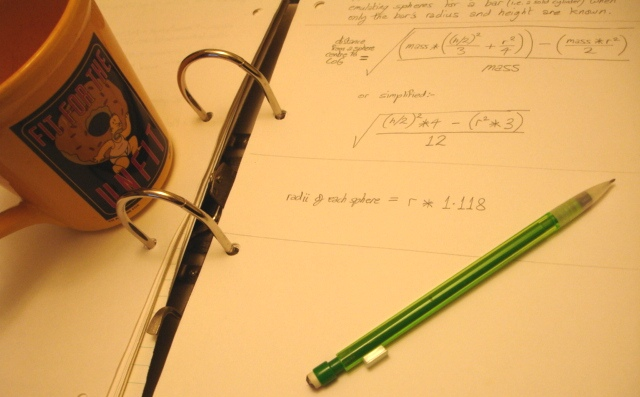# Jon's Video Game Programming Notes.Introduction.

Hi folks. These notes and algorithms are ones I have made for my own purposes in the past. I've posted them here incase they may be of use or interest to others. But now I'm also starting to write some notes for new-commers to video game programming ( the first of which is on simple collision detection ).

Most of these are notes on geometry in the context of programming video games. Some of them won't describe good or even correct methods of geometry, but instead describe dirty short cuts to arrive at quick approximations.

There are many ways to skin a cat, so don't assume any of these notes describe the only way to arrive at a solution, it is best to look at a few different methods, from different sources, and choose the one most efficient for your programming problem, perhaps even try benchmarking them in your target build.

Sorry for the poor repoduction and low contrast of some of the notes and diagrams (which were in pencil). I'll draw them-up on CAD or something when I get time.

First are listed some of my notes and then, further down the page, some of my algorithms (most are written in C).

Feel free to use anything on this page in your own games... if you find these notes or algorithms really useful for your game or project perhaps put my name in the credits... right down the bottom in teeny tiny small print will do fine. :-)

If you need to ask any questions, or you want ot say hi, please drop me an email. My email address.

# NOTES

COLLISION DETECTION, ETC.
Simple 2D collision Detection.
Find the Closest Points Between two 4D Lines. (NOTES10#)
Useful for collision detection with high-speed objects (if you consider the paths of two objects as two four dimensional lines). Use in conjunction with NOTES4# below if your collision objects are spheres or circles on linear paths.
Calculate Where Two Spheres or Circles Collide. (NOTES4#)
If you know where along the travel of two spheres or circles they come closest to each other (for example by using the method NOTES10# above) then you can use this approximation method to find where they actually collide.
Determine Which of a Number of Points an Object Will Pivot On (NOTES5#)

MATRICES.
Step-by-Step Illustration of 3x3 Matrix Multiplication. (NOTES15#)
An Illustration of Applying a Matrix to a Vector to Transform it. (NOTES16#)
(Using a 3x3 matrix plus a translation row).
Illustration of Trigonomic Matrix Multiplication. (Notes17# and Notes18#).
Also see how sine and cosine are use in rotation matrices.

MECHANICS.
Rough 'Ball Park' Figures for the Moment of Inertia of a Person. (NOTES19#)
A Fully Rotatable and Translatable Moment of Inertia Calculation System. NOTES14#
How to emulate any object's MoI with spheres so that the MoI can be rotated and translated up or down a complex hierachy of co-ordinate systems -- for example, for automatically calculating the MoI of a human body with its limbs in any position with the advantage of being able to use the same kind of transformation matrices that you would use to produce the graphics.

MISCELANEOUS.
Sphere Quandrants (NOTES13#)
(Reference for source code only -- not for viewing in its own right).

auto_arc_tan() (v1) Find the angle of a 2D line. Integer Version. (Uses an arctan emulation formula)
This does a similar job to the standard C arctan2() function but if you're program uses integer arithmatic (instead of floating point) then you may not have the equivelent integer function... so here is one you could use. I've put a lot of comments in this version to make it easy to understand.

auto_arc_tan() (v5) Find the angle of a 2D line. Integer Version. (Uses an arctan look-up table)
Same as the above routine, but it uses a look-up table rather than a formula to get the arc tangent values.

sine_12() Find the sine and or cosine. Integer Version.
A simple routine that looks-up a sine and or cosine for an angle from a table of figures.

distance_2D() and length_2D() Integer Version. (Uses a look-up table).
Find the distance between two 2D co-ordinates, or find the length of a 2D line (a vector).

fp_distance() and fp_length() Floating point Version. (Uses a emulation formula).
Find the distance between two 3D co-ordinates, or find the length of a 3D line (a vector).

distance_to_chord() Find an unknown Non-Hypotenuse Length. Integer Version. (Uses a look-uptable).
Find the distance from the centre of a circle to the centre of a chord line by supplying the chord length and the circle radius.
Alternatively this could be though-of as finding the unknown length of a side of a right angled triangle when you know the opposite or adjacent side's length and the hypotenus's length.

extend_to_perpendicular() Make one vector perpendicular to an other. Integer version.
Make one vector perpendicular (at 90 degrees) to another in 3D space, preserving the original 2D plane that the original vectors formed.

Normally you supply an angle to a sine or cosine function... but if you only know the gradient you can save time by not having to calculate the angle first and use these functions instead.

rotate_2D_with_sine_and_cosine(). Rotate a Vector. Integer Version.
Rotate a 2D vector ( or rotate a 3D vector about a single axis ) without using matrices.

rotate_vector(). Rotate a Vector. Floaing Point Version.
Rotate a 3D vector about a single axis without using matrices.

squared_chord_length_to_angle(). Integer Version. (Uses Emulation Formulae)
Find the angle between two vectors in 3D space.... or in other words find the apex angle of an isosceles triangle.

Convert To and From Big Number Bases.
You can use this program to convert a large integer number to a shorter, easier to remember, alpha-numeric figure.

A Simple Bouncing Ball Program.
A program that might be interesting to beginers. It is written in the freely available Yabasic language.

Email me.

HOME